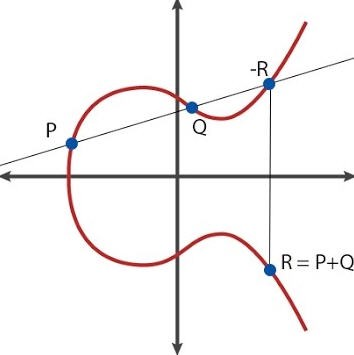## Generating Ethereum address from scratch

In this blog post I would like to introduce readers into Elliptic Curve Cryptography and use this knowledge to construct an Ethereum address.

# Part 1: Elliptic Curves

An Elliptic Curve is a curve define by the equation

$y^2 = x^3 + ax +b,$

The curve has a number of nice properties, and one of them is that if take two points on the curve and draw a line through them, the line will intersect the curve at exactly one point. You can play around with this property using the Bokeh application below:

CODE

This property allows us to map 2 points on a curve to a 3 point, an operation that we will refer to as an addition (with respect to the curve). The reason we call this an addition, because it shares certain similarities with an arithmetic addition. However, we refine our addition to result in the reflected point, see illustration below:Algebraically, this means that

\begin{align} \begin{split} x_R &= s^2 - x_P - x_Q, \\ y_R &= y_P + s(x_R - x_P) . \end{split} \tag{1}\label{eqn:point_r} \end{align}

where slope, $$s$$, defined as

$\frac{y_P - y_Q}{x_P - x_Q},$

when $$P \neq Q$$, and

$\frac{3x_P^2 + a}{2y_P},$

when $$P = Q$$. Note that in this case the slope is just a tangent and is easy to derive:

$\frac{\partial (y^2)}{\partial x} = \frac{\partial (x^3 + ax +b)}{\partial x} \implies \\ 2y \frac{\partial y}{\partial x} = 3x^2 + a.$

We can verify algebraically that $$y_R^2 = x_R^3 + ax_R + b$$, meaning $$R$$ is on our curve. Finally, for our addition to be valid, we need to define a point that would serve the role of $$0$$. So, by definition, $$P + O = P$$, and $$-P + P = O$$. My vague intuition is that I can think of $$O$$ as the entire x-axis.

# Part 2: Mathematical Fields

If we pick a prime number $$p$$, we can define multiplication, addition, subtraction and division module $$p$$:

Mathematically, this means that we have a field, $$\mathbb{F}_p$$. Real numbers form a field too, so you probably have a good intuition for how fields work.

Here are arithmetic tables for $$\mathbb{F}_{7}$$, skipping subtraction as I don’t have space:

*1 2 3 4 5 6
11 2 3 4 5 6
22 4 6 1 3 5
33 6 2 5 1 4
44 1 5 2 6 3
55 3 1 6 4 2
66 5 4 3 2 1
/1 2 3 4 5 6
11 2 3 4 5 6
24 1 5 2 6 3
35 3 1 6 4 2
42 4 6 1 3 5
53 6 2 5 1 4
66 5 4 3 2 1
+1 2 3 4 5 6
12 3 4 5 6 0
23 4 5 6 0 1
34 5 6 0 1 2
45 6 0 1 2 3
56 0 1 2 3 4
60 1 2 3 4 5

Plot twist: we are now going to deal with an elliptic curve defined over $$\mathbb{F}_p$$. Turns out the equations for addition with respect to a curve, \eqref{eqn:point_r}, are still well-defined: they only use simple arithmetic operations (*, /, +, -) anyway. Unfortunately, we no longer have the geometric intuition, as our curve plotted will now look like just a bunch of points.

# Part 3: Toy Elliptic Curve over $$\mathbb{F}_{37}$$

I wrote some auxiliary code that implements fields and elliptic curves, attached in appendix. For now, let’s use play with it to get used to the elliptic curves over prime fields:

I started with a point $$(0, 4)$$, module 37, and kept adding it until I reached $$O$$. I have to reach $$O$$, because the number of points on the curve is finite, so they have to start repeating:

$nP = mP \implies (n-m)P = 0,$

for some $$n \neq m$$. Are there any more points on the curve? Let’s find out:

Yes, there’s at least one more point: $$(1, 18)$$. Any more?

Looks like $$(1, 18)$$ generates all points on our curve. How many points do we have? 49:

In mathematics , we say that the order of point2 is 49, whilst the order of point1 is 7, where order is the number of times we add until we reach zero. point2 in this toy example plays the same role that number $$1$$ plays in integers: by adding $$1$$ repeatedly we an generate all positive integers. Same holds for point2: by adding it repeatedly we generate all points on the curve. OK, hopefully you get a better feel for this object. Let’s proceed.

# Part 4: Ethereum Address

I went on vanity-eth.tk and generated a public and a private key:

We have already covered all the material needed to replicate such computation. Both Ethereum and Bitcoin use an elliptic curve $$y^2 = x^3 + 7$$ over $$\mathbb{F}_p$$, where $$p = 2^{256} - 2^{32} - 2^{9} - 2^{8} - 2^{7} - 2^{6} - 2^{4} - 1$$, a very large prime.

It turns out whilst calculating $$n * P = Q$$, i.e. summing $$P$$ with itself $$n$$-times, is computationally easy, finding $$n$$, given $$P$$ and $$Q$$ is computationally hard. So the private key is $$n$$, whilst the public key is $$n * P$$, for some specified $$P$$. Below is the code that defines the curve and the specified point, taken from the secp256k1 specs:

Finally, we can generate the public point using multiplication:

That’s almost our public key, but not quite. We now encode (x, y) coordinates of our public point in hex, to get something like

Finally, we compute Keccak-256 hash function on the public point, and take last 40 characters:

Voilà!

# Credits

Thanks to bellbind github account for his toy implementations and Timur Badretdinov for his blog post.

ALL THE CODE